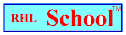Name_______________________________________________Date_________________________
Research Skills
Volume 5, Number 15, January 10, 2000
www.rhlschool.com

Mathematics Terms

If you are unsure of the meaning of any math term, look up the underlined word in a dictionary or math glossary.

1. Which of the following is an example of an integer?

a. half of any odd number
b. \$25.32
c. 1/10
d. 82

2. Which of the following are congruent?

a. two index cards: They are the same shape and size.One is white; one is blue.
b. any two objects that touch each other
c. a red cone and a red cube
d. two squares: One is exactly twice the size of the other.

3. Which of the following is an extrapolation?

a. I own half of a business that made 10 million dollars so I get 5 million dollars.
b. There are too many objects in the box. We are removing some of them until we reach the correct amount.
c. Every year my business makes twice as much money as the year before. The business made \$4000 in 1999 so I expect it to make \$8000 in 2000.
d. We will put the box on a scale in order to weigh it. Then we’ll subtract ten pounds because the scale always reads ten pounds too high.

4. In 34 = 3 X 3 X 3 X 3 = 81, which is the exponent?

a. 3
b. 4
c. 81
d. X

rhlschool.comRHL School - Free Worksheets and MoreCopyright 2000 RHL

Our Source: Harcourt Brace Math Glossary: http://www.hbschool.com/glossary/math/